﻿ Paradox Algorithm in Application of a Linear Transportation Problem

### Paradox Algorithm in Application of a Linear Transportation Problem

Osuji George A., Opara Jude, Nwobi Anderson C., Onyeze Vitus, Iheagwara Andrew I.OPEN ACCESSPEER-REVIEWED

## Paradox Algorithm in Application of a Linear Transportation Problem

Osuji George A.1, Opara Jude2, Nwobi Anderson C.3, Onyeze Vitus2, Iheagwara Andrew I.4

1Department of Statistics, Nnamdi Azikiwe University, Awka Anambra State Nigeria

2Department of Statistics, Imo State University, Owerri Nigeria

3Department of Statistics, Abia State Polytechnic, Aba Nigeria

4Department of Planning, Research and Statistics, Ministry of Petroleum and Environment Owerri Imo State Nigeria

### Abstract

Paradox seldom occurs in a linear transportation problem, but it is related to the classical transportation problem. For specific reasons of this problem, an increase in the quantity of goods or number of passengers (as used in this paper) to be transported may lead to a decrease in the optimal total transportation cost. Two numerical examples were used for the study. In this paper, an efficient algorithm for solving a linear programming problem was explicitly discussed, and it was concluded that paradox does not exist in the first set of data, while paradox exists in the second set of data. The Vogel’s Approximation Method (VAM) was used to obtain the initial basic feasible solution via the Statistical Software Package known as TORA. The first set of data revealed that paradox does not exist, while the second set of data showed that paradox exists. The method however gives a step by step development of the solution procedure for finding all the paradoxical pair in the second set of data.

• A., Osuji George, et al. "Paradox Algorithm in Application of a Linear Transportation Problem." American Journal of Applied Mathematics and Statistics 2.1 (2014): 10-15.
• A., O. G. , Jude, O. , C., N. A. , Vitus, O. , & I., I. A. (2014). Paradox Algorithm in Application of a Linear Transportation Problem. American Journal of Applied Mathematics and Statistics, 2(1), 10-15.
• A., Osuji George, Opara Jude, Nwobi Anderson C., Onyeze Vitus, and Iheagwara Andrew I.. "Paradox Algorithm in Application of a Linear Transportation Problem." American Journal of Applied Mathematics and Statistics 2, no. 1 (2014): 10-15.

 Import into BibTeX Import into EndNote Import into RefMan Import into RefWorks

### 1. Introduction

Paradox occurs when a transportation problem admits of a total cost which is lower than the optimum and is attainable by shipping larger quantities of goods over the same routes that were previously designated as optimal. This phenomenon which does not occur regularly was discovered by Szwarc (1971). It is obvious that many researchers have discovered independently from each other the following behavior of the transportation problem. In certain cases of the Transportation Problem (TP), an increase in the supplies and demands may lead to a decrease in the optimal transportation cost. In other words, by moving bigger amount of goods around, one may save a lot of money. This surely sounds paradoxical (Deineko, et al.; 2003).

The classical transportation problem is the name of a mathematical model which has a special mathematical structure. The mathematical formulation of a large number of problems conforms to this special structure. Hitchcock (1941) originally developed the basic linear transportation problem. Charnes et al (1953) developed the stepping stone methods which provide an alternative way of determining the simplex method information. Dantzig (1963) used the simplex method to the transportation problem as the primal simplex transportation method. Appa (1973) also developed the solution procedure for solving the transportation problem and its variants. Klingman and Russel (1974 and 1975) introduced a specialized method for solving a transportation problem with several additional linear constraints. Hadley (1987) gave the detailed solution procedure for solving linear transportation problem. Till date, several researchers studied extensively to solve cost minimizing transportation problem in various ways.

In some situations, if we obtain more flow with lesser cost than the flow corresponding to the optimum cost then we say paradox occurs. Charnes and Klingman (1971), Szwarc (1973), Adlakha and Kowalski (1998) and Storoy (2007) considered the paradoxical transportation problem. Gupta et al (1993) considered a paradox in linear fractional transportation problem with mixed constraints. Joshi and Gupta (2010) studied paradox in linear plus fractional transportation problem. In the early day of linear programming problem some of the pioneers observed paradox but by whom no one knows.

In this paper we present a method for solving transportation problem with linear constraints. Thereby, we state the sufficient condition of existence of paradox, paradoxical range of flow and paradoxical flow for a specified flow in such type of linear transportation problem. We also justify the theory by illustrating a numerical example.

### 2. Definitions

1. Paradox in a transportation problem: in a transportation problem if we can obtain more flow (F1) with lesser cost (Z1) than the optimum flow (F0) corresponding to the optimum cost (Z0) i.e. F1 > F0 and Z1 < Z0, then we say that a paradox occurs in a transportation problem.

2. Cost-flow pair: if the value of the objective function is Z0 and the flow is F0 corresponding to the feasible solution X0 of a transportation problem, then the pair (Z0, F0) is called the cost-flow pair corresponding to the feasible solution X0.

3. Paradoxical pair: A cost-flow pair, (Z, F) of an objective function is called paradoxical pair if Z < Z0 and F > F0 where Z0 is the optimum cost and F0 is the optimum flow of the transportation problem.

4. Best paradoxical pair: The paradoxical pair (Z*, F*) is called the best paradoxical pair of a transportation problem if for all paradoxical pair (Z, F), either Z* < Z or Z* = Z but F* > F.

5. Paradoxical range of flow: if F0 be the optimum flow and F* be the flow corresponding to the best paradoxical pair of a transportation problem then [F0, F*] is called paradoxical range of flow.

### 3. Review of Related Literature

The transportation paradox is, however, hardly mentioned at all in any of the great number of textbooks and teaching materials where the transportation problem is treated. Apparently, several researchers have discovered the paradox independently from each other. But most papers on the subject refer to the paper by Charines and Klingman (1971) and Szwarc (1971) as the initial papers. In Charines and Klingman (1971) name it the more-for-less paradox and they write: “The paradox was first observed in the early days of linear programming history (by whom no one knows) and has been part of the folklore known to some (e.g. A. Charnes and Cooper; 1953) but unknown to the great majority of workers in the field of linear programming.

Arora and Ahuja (2010) carried out a research work in paradox on a fixed charge transportation problem. In their findings, a paradox arises when the fixed charge transportation problem admits of a total cost which is lower than the optimum cost, by transporting larger quantities of goods over the same routes. A sufficient condition for the existence of a paradox is established. Paradoxical Range of flow is obtained for any given flow in which the corresponding objective function value is less than the optimum value of the fixed charge transportation problem.

Ekezie et al (2013) carried out a research on the determination of paradoxical pairs in a linear transportation problem. In their study, an efficient algorithm for solving a linear programming problem was discussed, and it was concluded that paradox exists. The North-West Corner method was used to obtain the initial basic feasible solution for the optimal solution. They also used the algorithm discussed to develop a step by step solution procedure for finding all the paradoxical pairs.

According to Appa (1973), the transportation paradox is known as Doigs paradox at the London School of Economics, named after Alison Doig who used it in exams etc around 1959 (Doig did not publish any paper on it).

Since the transportation paradox seems not to be known to the majority of those who are working with (or teaching) the transportation problem, one may be tempted to believe that this phenomenon is only an academic curiosity which will most probably not occur in any practical situation. But that seems not to be true. Experiments done by Finke (1978), with randomly generated instances of the transportation problem of size 100 100 and allowing additional shipments (post-optimal) show that the paradoxical properties. More precisely, the average cost reductions achieved are reported to be 18.6% with total additional shipments of 20.5%.

Ekezie et al (2013) carried out a research on the Paradox sum of a linear and a linear fractional transportation problem using data that were collected from a secondary source. In the study, a transportation problem with an objective function as the sum of a linear and linear fractional function was considered. A paradoxical situation arises in the sum of a linear and linear fractional transportation problem, when value of the objective function falls below the optimal value and this lower value is attainable by transporting larger number of passengers. An algorithm is proposed for finding initial basic feasible solution for the sum of a linear and linear fraction transportation problem and a sufficient condition for the existence of a paradoxical solution is established.

In a recent paper (2003), Deineko et al developed necessary and sufficient conditions for a cost matrix C to be immune against the transportation paradox. As well see in the next section, these conditions are rather restrictive, supporting the observations by Finke.

### 4. Problem Formulation

In this paper, we consider the following transportation problem:

Let the transportation problem consists of m sources and n destinations, where

xij = the amount of product transported from the ith source to the jth destination,

cij = the cost involved in transporting per unit product from the ith source to the jth destination,

ai = the number of units available at the ith source,

bj = the number of units required at the jth destination.

In this paper, we consider the cost minimizing linear transportation problem as: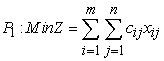subject to the constraints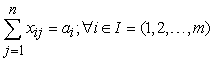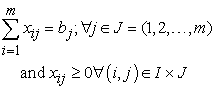Let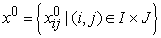be a basic feasible solution corresponding to the basis B of the problem P1 and the value of the objective function Z0 corresponding to the basic feasible solution X0 is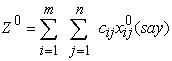Let F0 be the corresponding flow.

Then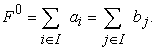Now we consider the dual variables ui for i ∈ I and j ∈ I such that ui + vj = cij corresponding to the basis B.

Also ∀(I, j)∉B, let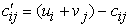If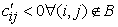then the solution is optimum.

Theorem: the sufficient condition for the existence of paradoxical solution of (P1) is that if ∃ at least one cell (r, s) ∉ B in the optimum table of (P1) where ar and bs are replaced by ar + l and bs + l respectively (l > 0) then (ur + vs) < 0.

Proof: Let Z0 be the value of the objective function and F0 be the optimum flow corresponding to the optimum solution (X0) of problem (P1). The dual variables ui and vj are given by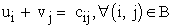Then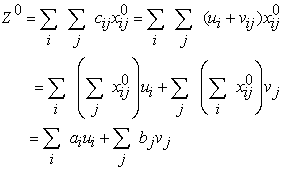and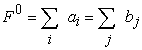Now, let ∃ at least one cell (r, s)∉B, where ar are replaced by ar + l and bs + l, respectively (l > 0) in such a way that the optimum basis remains same, then the value of the objective function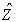is given by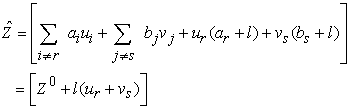The new flow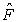is given by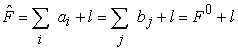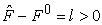Therefore for the existence of paradox we must have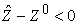. Hence the sufficient condition for the existence of paradox is that ∃ at least one cell (r, s)∉B in the optimum table of such that if ar and bs are replaced by ar + l and bs + l (l > 0) then l( + vs) < 0, i.e. (ur + vs) < 0.

Now we state the following algorithm to find all the paradoxical pair of the problem (P1).

Algorithm:

Step 1: Find the cost-flow pair (Z0, F0) for the optimum solution X0.

Step 2: i = 1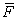Step 3: Find all cells (r, s)∉B such that (ur + vs) < 0 if it exists otherwise go to step 8.

Step 4: Among all cells (r, s) ∉B satisfying step 3 find min flow for l = 1 which enter into the existing basis whose corresponding cost is minimum. Let (Zi, Fi) be the new cost flow pair corresponding to the optimum solution Xi.

Step 5: Write (Zi, Fi).

Step 6: i = i + 1.

Step 7: go to step 3

Step 8: We write the best paradoxical pair (Z*, F*) = (Zi, Fi) for the optimum solution X* = Xi.

Step 9: End.

This algorithm gives all the paradoxical pairs. From these pairs we can find the paradoxical pair for a specified flow () also.

### 5. Data Analysis

In this paper, we shall use three sets of data to enable us achieve our primary aim. Firstly, we shall examine the data gotten from ABC Transport Company, owerri . ABC Transport Company Ltd has many types of buses that ply different routes in . In this study, we shall restrict the work to only 6 different types of buses that ply 4 different routes in . Table 1 shows the expected number of passengers each type of bus can carry in a day, and the cost of transporting a passenger from Owerri to the different routes in Nigeria by each of the buses.

#### Table 1.

Solving the transportation problem in Table 1, the result is displayed in Table 2.

The total cost of transportation is N2, 454,440. We then check the sign of (Ur + Vs), where (r, s) ∉ B in Table 2, we observe that all the Ui’s + Vj’s > 0. With this result, we can conclude that paradox does not exist, which implies that the algorithm discussed in this paper will be irrelevant to this set of data.

Let us now consider the second set of data used for this research, which was extracted from Opara (2009), Introduction to Operation Research, Exercises 3 page 28. The estimated supply capacities of the six warehouses, the demand requirements at the seven markets and the transportation cost of each product are given in Table 3.

Solving the above problem using the Vogel’s Approximation Method (VAM), the optimal transportation table is presented in Table 4.

The total cost is 759. We then check the sign of (Ur + Vs), where (r, s) ∉ B in Table 4, we observe that U5 + V3 = -3 < 0, and U5 + V5 = - 1 < 0. This implies that Paradoxical pair of the problem (P1) exists. So applying the algorithm discussed in this paper, we have;

Applying Step 1: The cost-flow pair is (Zo, Fo) = (759, 173) corresponding to the optimum solution X = {x12 = 13, x13 = 34, x14 = 13, x22 = 16, x25 = 17, x26 = 6, x31 = 26, x34 = 7, x41 = 20, x52 = 3, x61 = 3, x67 = 5}.

Applying step 2: set i=1

Applying step 3: Now we check the sign of (Ur + Vs) and we obtain for the non-basic cells (5, 3) and (5, 5), the sign that is negative.

Applying step 4: For l = 1

For the cell (5, 3)

The total cost of this transportation problem is 756.

For cell (5, 5), the transportation table is presented in Table 6.

The total cost of this transportation problem is 758.

The min cost = min{756, 758} = 756.

Hence l = 1 enters in the optimum basis from the cell (5, 3) and corresponding table is Table 5, the corresponding paradoxical pair (Z′, F′) = (756, 174).

Employing steps 6 and 7. Then repeating this process, the next table is

Now repeating this process, the final table is presented in Table 8.

Hence, from the above table, the corresponding paradoxical pair (Z′, F′) = (720, 186).

Applying step 8: The best paradoxical pair is (Z*, F*) = (720, 186) corresponding to the optimum solution is X* = {x12 = 0; x13 = 47, x14 = 13, x22 = 16, x25 = 17, x26 = 6, x31 = 26, x34 = 7, x41 = 20, x52 = 26, x61 = 3, x67 = 5} and the paradoxical range of flow is [F0, F*] = [173, 186]. Thus, all the paradoxical pairs are {(756, 174), (753, 175), (750, 176), (747, 177), (744, 178), (741, 179), (738, 180), (735, 181), (732, 182), (729, 183), (726, 184), (723, 185) and (720, 186).

Let us consider the third set of data from this hypothetical example for more clarification of the algorithm. The estimated supply capacities of the five warehouses, the demand requirements at the five markets and the transportation cost of each product are given in Table 8.

Solving the above problem using VAM via TORA Statistical Software Package, the optimal transportation table is presented in Table 10.

The total cost is 320. Again, we then check the sign of (Ur + Vs), where (r, s) ∉ B in Table 10, we observe that U2 + V5 = -2 < 0, U3 + V5 = - 1 < 0, and U4 + V5 = - 1 < 0. So, paradoxical pairs exist.

Applying Step 1: The cost-flow pair is (Zo, Fo) = (320, 142) corresponding to the optimum solution X = {x11 = 6, x12 = 26, x14 = 3, x15 = 10, x21 = 35, x31 = 3, x33 = 27, x44 = 22, x52 = 10}.

Applying step 2: set i=1

Applying step 3: Now we check the sign of (Ur + Vs) and we obtain for the non-basic cells (2,5), (3,5) and (4,5), the sign that is negative.

Applying step 4: For l = 1

For the cell (2, 5)

The total cost of this transportation problem is 318.

For cell (3, 5), the transportation tableau is presented in Table 12.

The total cost of this transportation problem is 319.

For the cell (4, 5), the transportation tableau is presented in Table 13.

The total cost is 319.

The min cost = min {318, 319, 319} = 318.

Hence l = 1 enters in the optimum basis from the cell (2, 5) and corresponding table is Table 10, the corresponding paradoxical pair (Z′, F′) = (318, 143).

Employing steps 6 and 7. Then repeating this process again, the next tableau is

Now repeating this process, the final table is presented in Table 15.

#### Table 15.

Hence, from the above table, the corresponding paradoxical pair (Z′, F′) = (308, 148).

Applying step 8: The best paradoxical pair is (Z*, F*) = (308, 148) corresponding to the optimum solution is X* = {x11 = 0; x12 = 26, x14 = 3, x15 = 16, x21 = 41, x31 = 3, x33 = 27, x44 = 22, x52 = 10} and the paradoxical range of flow is [F0, F*] = [142, 148]. Thus, all the paradoxical pair are {(318, 143), (316, 144), (314, 145), (312, 146), (310, 147), and (308, 148)}.

### 6. Conclusion

In this paper, an attempt has been made to discuss an efficient statistical algorithm for computing paradox in a linear transportation problem if paradox does exist. The algorithm gives step by step development of the solution procedure for finding all the paradoxical pair where paradox exists, well understanding. The statistical software package known as “TORA” was used to obtain the optimal solution before adopting the algorithm of paradoxical pairs.

Future research should be done on a related work like in this paper, and the author should try to develop a program that will enable one to obtain the best paradoxical pairs instead of solving it manually, and as well the paradoxical range of flow.

### References

  Adlakha V. and Kowalski, K. (1998): A quick sufficient solution to the more-for-less paradox in a transportation problem, Omega 26(4):541-547.In article CrossRef  Appa G.M. (1973): The Transportation problem and its variants, Oper. Res. Q. 24:79-99.In article  Arora S.R. and Ahuja A. (2000): A paradox in a fixed charge transportation problem. Indian J. pure appl. Math., 31(7): 809-822, July 2000 printed in India.In article  Charnes A.; Cooper W.W. and Henderson (1953): An Introduction to Linear programming (Wiley, New Work).In article  Charnes A. and Klingman D. (1971): The more-for-less paradox in the distribution model, Cachiers du Centre Etudes de Recherche Operaionelle 13; 11-22.In article  Deineko, V.G; Klinz, B and Woeginger, G.J. (2003): Which Matrices are Immune against the Transportation Paradox? Discrete Applied Mathematics, 130:495-501.In article CrossRef  Dantzig, G.D. (1963): Linear Programming and Extensive (Princeton University Press, NJ).In article  Dantzig G.B. (1951): Application of the simplex method to a transportation problem, in Activity Analysis of Production and Allocation (T.C. Koopmans, ed.) Wiley, New York, pp.359-373.In article  Ekezie, D.D.: Ogbonna, J.C. and Opara, J. (2013): The Determination of Paradoxical Pairs in a Linear Transportation Problem. International Journal of Mathematics and Statistics Studies. Vol. 1, No. 3, p.p.9-19, September 2013.In article  Finke, G.(1978): A unified approach to reshipment, overshipment and postoptimization problems, lecture notes in control and information science, Vol.7, Springer, Berlin, 1978, p.p.201-208.In article  Gupta, A. Khanna S and Puri, M.C. (1993): A paradox in linear fractional transportation problems with mixed constraints, Optimization 27:375-387.In article CrossRef  Hadley G. (1987): Linear Programming (Narosa Publishing House, New Delhi).In article  Hitchcock, F.L. (1941): The distribution of a product from several resources to numerous localities, J. Math. Phys. 20:224-230.In article  Joshi, V. D. and Gupta, N. (2010): On a paradox in linear plus fractional transportation problem, Mathematika 26(2):167-178.In article  Opara, J. (2009): Manual on Introduction to Operation Research, Unpublished.In article  Klingman, D. and Russel, R. (1974): The transportation problem with mixed constraints, operational research quarterly, Vol. 25, No. 3: p.p. 447-455.In article  Klingman, D. and Russel, R. (1975): Solving constrained transportation problems, Oper. Res. 23(1):91-105.In article CrossRef  Storoy, S. (2007): The transportation paradox revisited, N-5020 Bergen, Norway.In article  Szwarc W. (1971): The transportation paradox, Nav. Res. Logist. Q.18:185-202.In article CrossRef